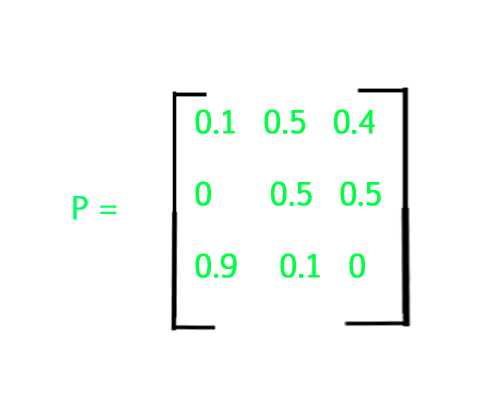# Program for Markov matrix

Given a m x n 2D matrix, check if it is a Markov Matrix.

Markov Matrix : The matrix in which the sum of each row is equal to 1.Example of Markov Matrix

Examples:

```Input :
1    0   0
0.5  0  0.5
0    0   1
Output : yes

Explanation :
Sum of each row results to 1,
therefore it is a Markov Matrix.

Input :
1 0 0
0 0 2
1 0 0
Output :
no
```

## Recommended: Please try your approach on {IDE} first, before moving on to the solution.

Approach : Initialize a 2D array, then take another single dimensional array to store the sum of each rows of the matrix, and check whether all the sum stored in this 1D array is equal to 1, if yes then it is Markov matrix else not.

## C++

 `// C++ code to check Markov Matrix ` `#include ` `using` `namespace` `std; ` ` `  `#define n 3 ` ` `  `bool` `checkMarkov(``double` `m[][n]) ` `{ ` `    ``// outer loop to access rows ` `    ``// and inner to access columns ` `    ``for` `(``int` `i = 0; i

## Java

 `// Java code to check Markov Matrix ` `import` `java.io.*; ` ` `  `public` `class` `markov ` `{ ` `    ``static` `boolean` `checkMarkov(``double` `m[][]) ` `    ``{ ` `        ``// outer loop to access rows ` `        ``// and inner to access columns ` `        ``for` `(``int` `i = ``0``; i < m.length; i++) { ` ` `  `            ``// Find sum of current row ` `            ``double` `sum = ``0``; ` `            ``for` `(``int` `j = ``0``; j < m[i].length; j++) ` `                ``sum = sum + m[i][j]; ` ` `  `            ``if` `(sum != ``1``) ` `               ``return` `false``; ` `        ``} ` ` `  `        ``return` `true``; ` `    ``} ` ` `  `    ``public` `static` `void` `main(String args[]) ` `    ``{ ` `        ``// Matrix to check ` `        ``double` `m[][] = { { ``0``, ``0``, ``1` `}, ` `                         ``{ ``0.5``, ``0``, ``0.5` `}, ` `                         ``{ ``1``, ``0``, ``0` `} }; ` ` `  `        ``// calls the function check() ` `        ``if` `(checkMarkov(m)) ` `            ``System.out.println(``" yes "``); ` `        ``else` `            ``System.out.println(``" no "``); ` `    ``} ` `} `

## Python3

 `# Python 3 code to check Markov Matrix ` ` `  `def` `checkMarkov(m) : ` `     `  `    ``# Outer loop to access rows ` `    ``# and inner to access columns ` `    ``for` `i ``in` `range``(``0``, ``len``(m)) : ` `         `  `        ``# Find sum of current row ` `        ``sm ``=` `0` `        ``for` `j ``in` `range``(``0``, ``len``(m[i])) : ` `            ``sm ``=` `sm ``+` `m[i][j] ` ` `  `        ``if` `(sm !``=` `1``) : ` `            ``return` `False` `             `  `    ``return` `True` `     `  `# Matrix to check ` `m ``=` `[ [ ``0``, ``0``, ``1` `], ` `      ``[ ``0.5``, ``0``, ``0.5` `], ` `      ``[ ``1``, ``0``, ``0` `]      ] ` ` `  `# Calls the function check() ` `if` `(checkMarkov(m)) : ` `    ``print``(``" yes "``) ` `else` `: ` `    ``print``(``" no "``) ` `     `  `     `  `# This code is contributed by Nikita Tiwari. `

## C#

 `// C# code to check  ` `// Markov Matrix ` `using` `System; ` ` `  `class` `GFG ` `{ ` `static` `bool` `checkMarkov(``double` `[,]m) ` `{ ` `    ``// outer loop to access  ` `    ``// rows and inner to  ` `    ``// access columns ` `    ``for` `(``int` `i = 0; ` `             ``i < m.GetLength(0); i++)  ` `    ``{ ` ` `  `        ``// Find sum of  ` `        ``// current row ` `        ``double` `sum = 0; ` `        ``for` `(``int` `j = 0;  ` `                 ``j < m.GetLength(1); j++) ` `            ``sum = sum + m[i, j]; ` ` `  `        ``if` `(sum != 1) ` `        ``return` `false``; ` `    ``} ` ` `  `    ``return` `true``; ` `} ` ` `  `// Driver Code ` `static` `void` `Main() ` `{ ` `    ``// Matrix to check ` `    ``double` `[,]m = ``new` `double``[,]{{ 0, 0, 1}, ` `                                ``{0.5, 0, 0.5}, ` `                                ``{1, 0, 0}}; ` ` `  `    ``// calls the  ` `    ``// function check() ` `    ``if` `(checkMarkov(m)) ` `        ``Console.WriteLine(``" yes "``); ` `    ``else` `        ``Console.WriteLine(``" no "``); ` `} ` `} ` ` `  `// This code is contributed by  ` `// Manish Shaw(manishshaw1) `

## PHP

 ` `

Output :

```yes
```

My Personal Notes arrow_drop_upCheck out this Author's contributed articles.

If you like GeeksforGeeks and would like to contribute, you can also write an article using contribute.geeksforgeeks.org or mail your article to contribute@geeksforgeeks.org. See your article appearing on the GeeksforGeeks main page and help other Geeks.

Please Improve this article if you find anything incorrect by clicking on the "Improve Article" button below.

Improved By : nitin mittal, manishshaw1

Article Tags :
Practice Tags :

2

Please write to us at contribute@geeksforgeeks.org to report any issue with the above content.